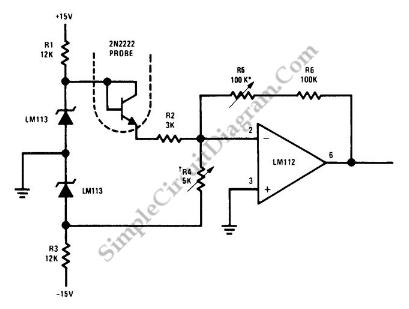# 2N222 Transistor As Temperature Sensor

This is a simple Electronic thermometer circuit. This is a inexpensive circuit because probe or sensor that is used in this circuit is 2N2222 silicon transistor. The accuracy of this circuit is better than 1°C over a 100°C range. The input voltage of this circuit is regulated to 1.2V by the LM113 diode. This voltage is used to set operating current of the temperature-sensing 2N2222. Here is the schematic diagram of the circuit:The feedback resistor R5 is used to calibrate the output scale factor to 100mV/°C. The amplifier output is biased for zero at 0°C by the R4. So, the scale factor does not change to zero when the output is zero. [Circuit’s schematic diagram source: seekic.com]